• 今天小编就为大家分享一篇python统计函数scipy.stats用法解析，具有很好参考价值，希望对大家有所帮助。一起跟随小编过来看看吧
• ## Python统计分析库statsmodels的OLS

万次阅读 多人点赞 2017-10-27 14:35:52
statsmodels官方文档http://www.statsmodels.org/stable/，里面包含很多统计模型和相应计算结果；比较有意思Linear Regression Models例子http://www.statsmodels.org/stable/examples/index.html#regression...
statsmodels库官方文档http://www.statsmodels.org/stable/，里面包含很多统计模型和相应计算结果；一些Linear Regression Models例子http://www.statsmodels.org/stable/examples/index.html#regression

下面主要陈述常用的回归分析中的OLS:Ordinary Least Squares。
给定k<!--//--><![CDATA[//><!--
k
//--><!]]>组样本数据(yi,x(i)1,x(i)2,⋯,x(i)n)，i=1,2,⋯,k<!--//--><![CDATA[//><!--
(y_{i},x_{1}^{(i)},x_{2}^{(i)},\cdots,x_{n}^{(i)})，i=1,2,\cdots,k
//--><!]]>，
用n+1<!--//--><![CDATA[//><!--
n+1
//--><!]]>维一次多项式回归模型 y(x)=α0+α1x1+α2x2+⋯+αnxn=(α0,α1,⋯,αn)⎛⎝⎜⎜⎜⎜⎜⎜⎜1x1x2⋮xn⎞⎠⎟⎟⎟⎟⎟⎟⎟≜αTx<!--//--><![CDATA[//><!--
y(x)=\alpha_{0}+\alpha_{1}x_{1}+\alpha_{2}x_{2}+\cdots+\alpha_{n}x_{n}\\
=(\alpha_{0},\alpha_{1},\cdots,\alpha_{n})\left( \begin{array}{ccc}
1\\
x_{1}\\
x_{2}\\
\vdots\\
x_{n}
\end{array}
\right )\triangleq\alpha^{T}x
//--><!]]>
其中α=(α0,α1,⋯,αn),x=(1,x1,x2,⋯,xn)T<!--//--><![CDATA[//><!--
\alpha=(\alpha_{0},\alpha_{1},\cdots,\alpha_{n}),x=(1,x_{1},x_{2},\cdots,x_{n})^{T}
//--><!]]>。

这里将常数项并入α,x<!--//--><![CDATA[//><!--
\alpha,x
//--><!]]>以增广形式表出是为了和statsmodels.OLS模块编写源码对应，OLS里的多项式回归模型是没有常数项的，所以这里将常数项看作基为1<!--//--><![CDATA[//><!--
1
//--><!]]>的维度上的系数，OLS就是用样本数据拟合出最小二乘最小的系数组合，即求α<!--//--><![CDATA[//><!--
\alpha
//--><!]]>。

对上述k<!--//--><![CDATA[//><!--
k
//--><!]]>组样本数据进行最小二乘拟合，即最小化 ∑i=1k(yi−α0+α1x(i)1+⋯+αnx(i)n)2<!--//--><![CDATA[//><!--
\sum_{i=1}^{k}(y_{i}-\alpha_{0}+\alpha_{1}x_{1}^{(i)}+\cdots+\alpha_{n}x_{n}^{(i)})^2
//--><!]]>statsmodels.OLS 的参数有endog, exog, missing, hasconst等 ，现在只考虑前两个。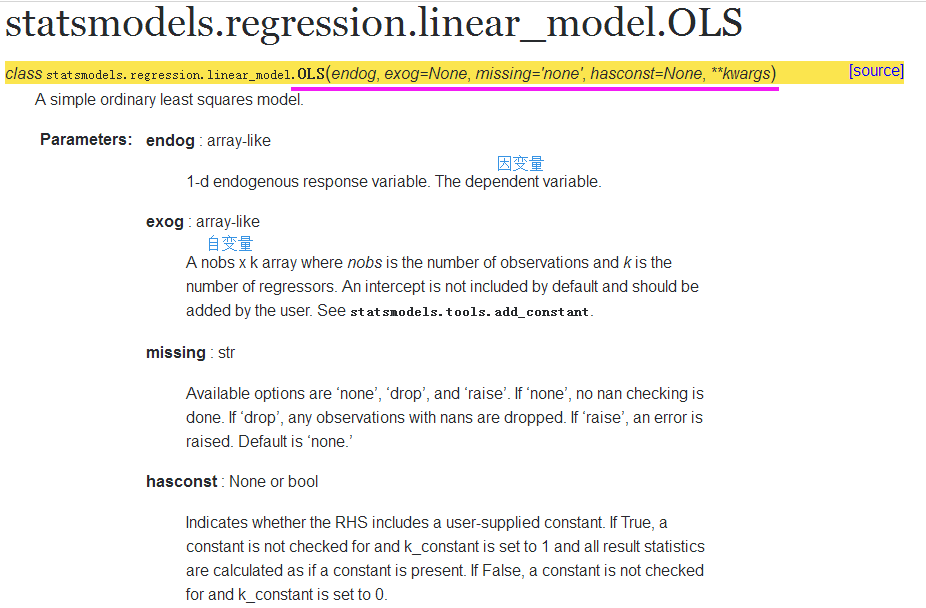第一个输入 endog 是回归模型中的因变量y(x)<!--//--><![CDATA[//><!--
y(x)
//--><!]]>, 输入是一个k<!--//--><![CDATA[//><!--
k
//--><!]]>维向量(y1,y2,⋯,yk)T<!--//--><![CDATA[//><!--
(y_{1},y_{2},\cdots,y_{k})^{T}
//--><!]]>。第二个输入 exog 是自变量，即k<!--//--><![CDATA[//><!--
k
//--><!]]>个样本点构成的k×(n+1)<!--//--><![CDATA[//><!--
k\times(n+1)
//--><!]]>维数组 ⎛⎝⎜⎜⎜⎜⎜⎜11⋮1x(1)1x(2)1⋮x(k)1x(1)2x(2)2⋮x(k)2⋯⋯⋱⋯x(1)nx(2)n⋮x(k)n⎞⎠⎟⎟⎟⎟⎟⎟<!--//--><![CDATA[//><!--
\left( \begin{array}{ccc}
1&x_{1}^{(1)}&x_{2}^{(1)}&\cdots&x_{n}^{(1)}\\
1&x_{1}^{(2)}&x_{2}^{(2)}&\cdots&x_{n}^{(2)}\\
\vdots&\vdots&\vdots&\ddots&\vdots\\
1&x_{1}^{(k)}&x_{2}^{(k)}&\cdots&x_{n}^{(k)}
\end{array}
\right )
//--><!]]>
通常，我们使用的数据集的k<!--//--><![CDATA[//><!--
k
//--><!]]>个样本点构成的数组第一列并不全是1<!--//--><![CDATA[//><!--
1
//--><!]]>，所以为了使用OLS模型函数，需要在数组左侧加上一列 1，就需要使用statmodels库的add_constant()函数，该函数的参数就是因变量数组(上述k×(n+1)<!--//--><![CDATA[//><!--
k\times(n+1)
//--><!]]>维数组去掉左侧一列1<!--//--><![CDATA[//><!--
1
//--><!]]>)，也就是数据分析中用到的具有物理含义的list、pd.Series、pd.DataFrame；该函数的输出就如上述形式的k×(n+1)<!--//--><![CDATA[//><!--
k\times(n+1)
//--><!]]>维数组。

import pandas as pd # 读取数据到DataFrame
import urllib # 获取网络数据
import shutil # 文件操作
import zipfile # 压缩解压
import os

# 建立临时目录
try:
os.system('mkdir bike_data')
except:
os.system('rm -rf bike_data; mkdir bike_data')

data_source = 'http://archive.ics.uci.edu/ml/machine-learning-databases/00275/Bike-Sharing-Dataset.zip' # 网络数据地址
zipname = 'bike_data/Bike-Sharing-Dataset.zip' # 拼接文件和路径
urllib.request.urlretrieve(data_source, zipname) # 获得数据

zip_ref = zipfile.ZipFile(zipname, 'r') # 创建一个ZipFile对象处理压缩文件
#zip_ref.extractall(temp_dir) # 解压
zip_ref.extractall('bike_data')
zip_ref.close()

daily_path = 'bike_data/day.csv'
daily_data = pd.read_csv(daily_path) # 读取csv文件
daily_data['dteday'] = pd.to_datetime(daily_data['dteday']) # 把字符串数据传换成日期数据
drop_list = ['instant', 'season', 'yr', 'mnth', 'holiday', 'workingday', 'weathersit', 'atemp', 'hum'] # 不关注的列
daily_data.drop(drop_list, inplace = True, axis = 1) # inplace=true在对象上直接操作

daily_data.head() # 看一看数据~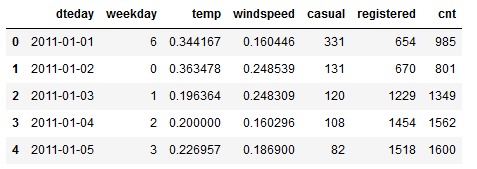import statsmodels.api as sm
#最小二乘
from statsmodels.stats.outliers_influence import summary_table
#获得汇总信息

x=sm.add_constant(daily_data['temp'])
#线性回归增加常数项 y=kx+b
y=daily_data['cnt']
regr=sm.OLS(y,x)
res=regr.fit() 

st, data, ss2 = summary_table(res, alpha=0.05)
#置信水平alpha=5%，st数据汇总，data数据详情，ss2数据列名
fitted_values = data[:,2]
#等价于res.fittedvalues

res.model.endog ==y.values
#拟合回归模型的endog值就是因变量y

res.fittedvalues  #获取拟合y值

res.params  #拟合回归模型参数
res.params+res.params*daily_data['temp']==res.fittedvalues  #验证二维回归模型的拟合y值计算原理

总结下，常用的OLS模型模板

import statsmodels.api as sm # 最小二乘
from statsmodels.stats.outliers_influence import summary_table # 获得汇总信息
x = sm.add_constant(daily_data['temp']) # 线性回归增加常数项 y=kx+b
y = daily_data['cnt']
regr = sm.OLS(y, x) # 普通最小二乘模型，ordinary least square model
res = regr.fit()    #res.model.endog
# 从模型获得拟合数据
st, data, ss2 = summary_table(res, alpha=0.05) # 置信水平alpha=5%，st数据汇总，data数据详情，ss2数据列名
fitted_values = data[:,2]  #等价于res.fittedvalues

以上内容参考statsmodels官网和pip install的源码
展开全文• ## statsmodels︱python常规统计模型库

千次阅读 多人点赞 2020-02-18 10:37:11
之前看sklearn线性模型没有R方，F检验，回归系数T检验等指标，于是看到了statsmodels这个，看着该输出结果真是够怀念。。 文章目录1 安装2 相关模型介绍2.1 线性模型2.2 离散选择模型(Discrete Choice ...
之前看sklearn线性模型没有R方，F检验，回归系数T检验等指标，于是看到了statsmodels这个库，看着该库输出的结果真是够怀念的。。

文章目录1 安装2 相关模型介绍2.1 线性模型2.2 离散选择模型(Discrete Choice Model, DCM)2.3 非参数统计2.4 广义线性模型 - Generalized Linear Models2.5 稳健回归——Robust Regression2.6 广义估计方程2.7 方差分析2.8 时间序列分析——Time Series Analysis2.9 空间计量必备：状态空间模型——State space models2.10 多元统计模型——因子/主成分分析3 相关模型demo3.1 线性回归模型3.2 广义线性模型——GLM3.3 稳健回归4 其他4.1 模型结果如何CSV导出？4.2 画模型图以及保存4.3 快速获取模型输出参数：P检验、F检验、P统计量

1 安装
pip install statsmodels

不过有可能会报错：
ImportError: cannot import name 'factorial' from 'scipy.misc'
(E:\Anaconda3.7\lib\site-packages\scipy\misc\__init__.py)


是跟scipy版本不匹配，笔者是删掉之前的pip uninstall statsmodels，再重新安装了一下就好了：
pip install --pre statsmodels -i https://pypi.tuna.tsinghua.edu.cn/simple

2 相关模型介绍
相关文档可见：https://www.statsmodels.org/stable/examples/index.html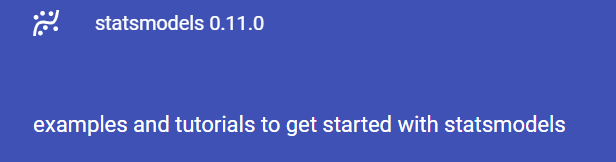包含的模型有：
2.1 线性模型2.2 离散选择模型(Discrete Choice Model, DCM)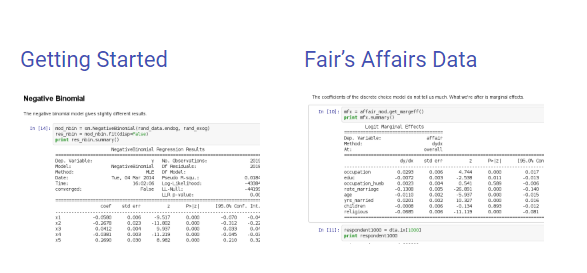参考：离散选择模型(Discrete Choice Model, DCM)简介——之一
离散选择模型（Discrete Choice Model, DCM）在经济学领域和社会学领域都有广泛的应用。
例如，消费者在购买汽车的时候通常会比较几个不同的品牌，如福特、本田、大众，等等。
如果将消费者选择福特汽车记为Y=1，选择本田汽车记为Y=2，选择大众汽车记为Y=3；那么在研究消费者选择何种汽车品牌的时候，由于因变量不是一个连续的变量（Y=1, 2, 3），传统的线性回归模型就有一定的局限（见DCM系列文章第2篇）。
再比如，在交通安全研究领域，通常将交通事故的严重程度划分为3大类：

（1）仅财产损失（Property Damage Only, PDO），
（2）受伤（Injury），
（3）死亡（Fatality）；
在研究各类因素（如道路坡度、弯道曲率等、车龄、光照、天气条件等）对事故严重程度的影响的时候，由于因变量（事故严重程度）是一个离散变量（仅3个选项），使用离散选择模型可以提供一个有效的建模途径。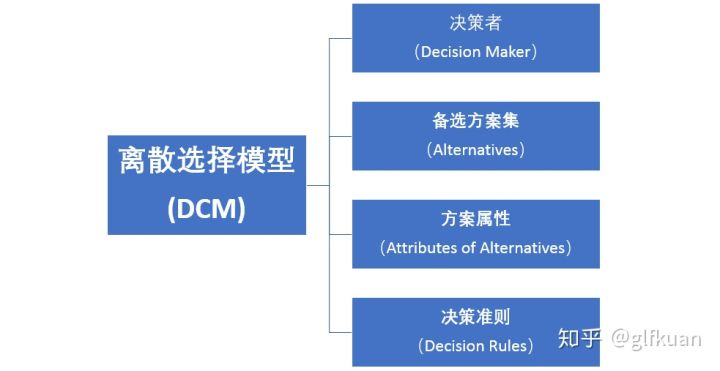2.3 非参数统计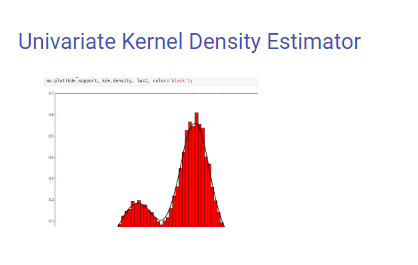2.4 广义线性模型 - Generalized Linear Models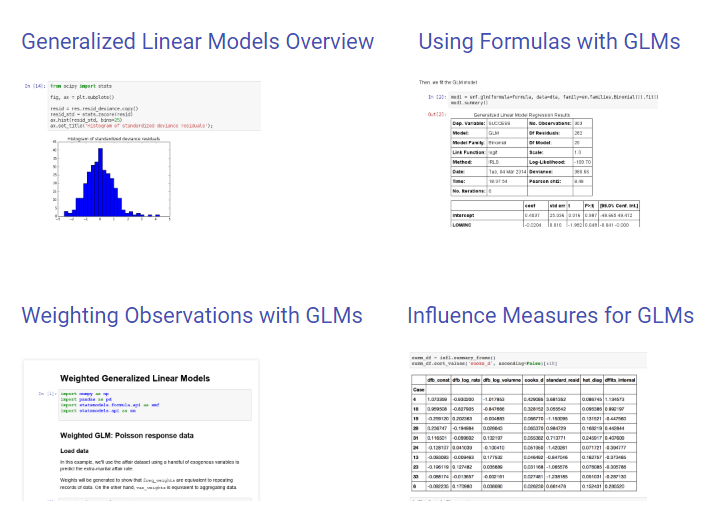2.5 稳健回归——Robust Regression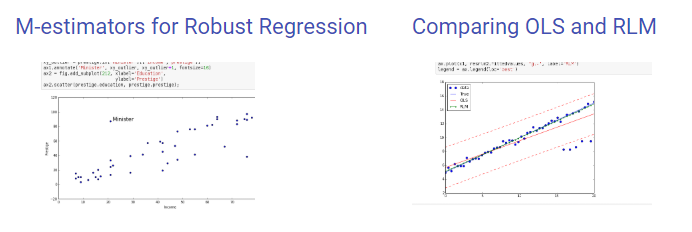2.6 广义估计方程2.7 方差分析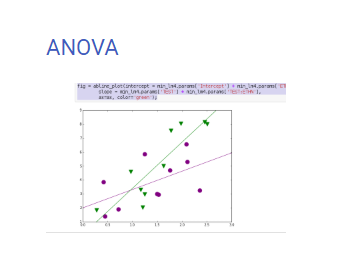2.8 时间序列分析——Time Series Analysis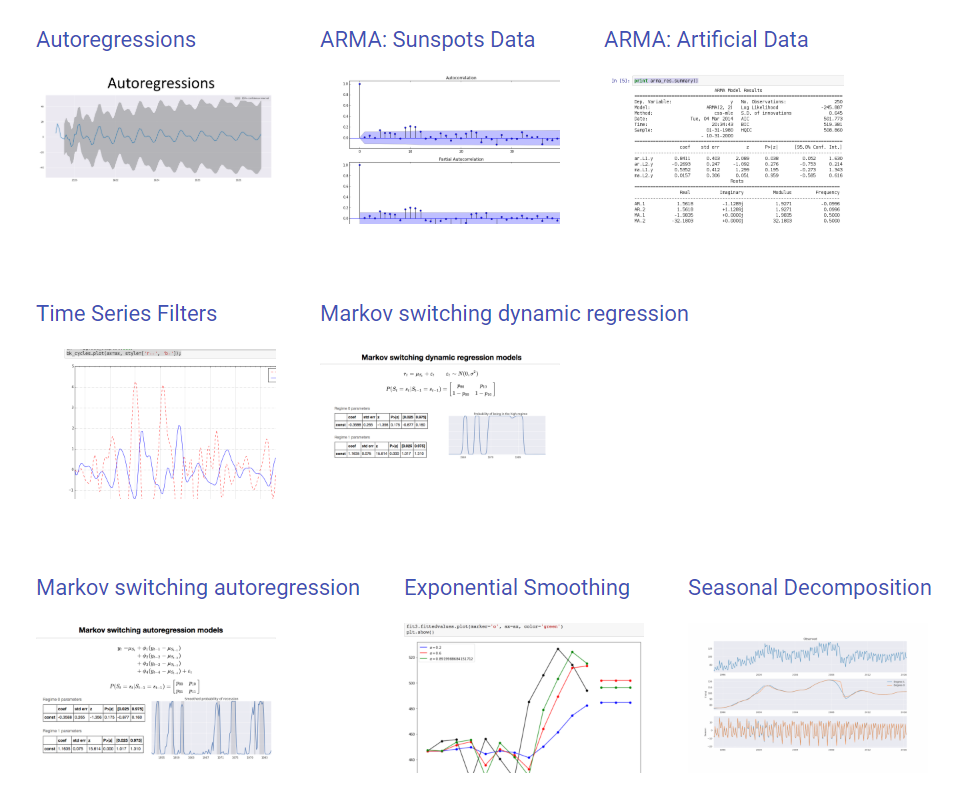2.9 空间计量必备：状态空间模型——State space models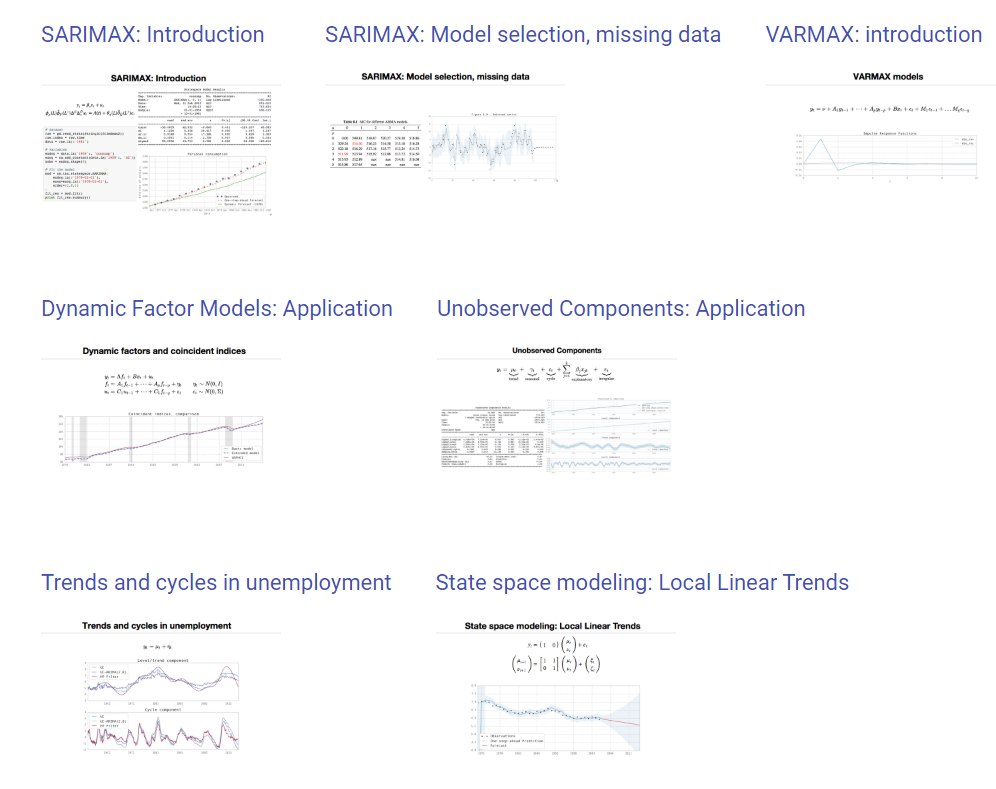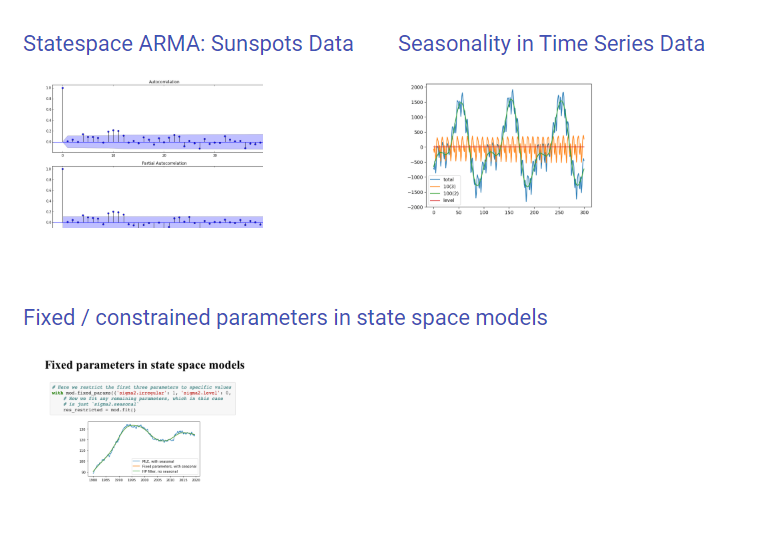2.10 多元统计模型——因子/主成分分析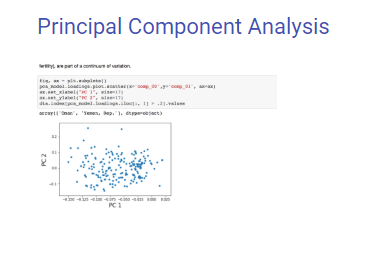3 相关模型demo
3.1 线性回归模型
可参考：https://www.statsmodels.org/stable/examples/notebooks/generated/ols.html
#  线性模型
import statsmodels.api as sm
import numpy as np
x = np.linspace(0,10,100)
y = 3*x + np.random.randn()+ 10
# Fit and summarize OLS model
X = sm.add_constant(x)
mod = sm.OLS(y,X)
result = mod.fit()
print('Parameters: ', result .params)
print('Standard errors: ', result .bse)
print('Predicted values: ', result .predict())
print(result.summary())

# 预测数据
print(result.predict(X[:5]))

输出结果超级熟悉。

result.params是回归系数
result.summary()把模型相关系数都打印出来
其中，预测的时候，如果不给入参数result.predict()，则默认是X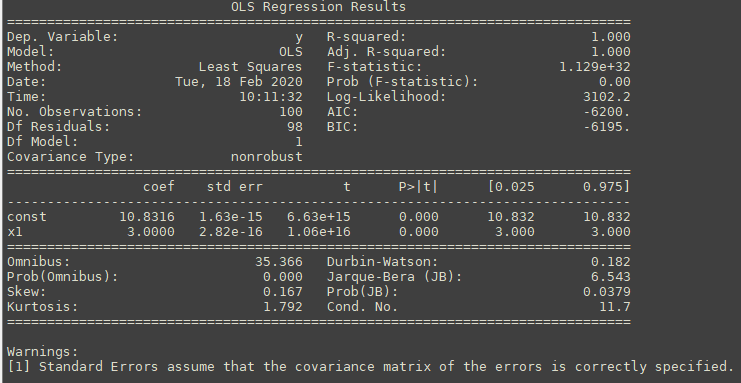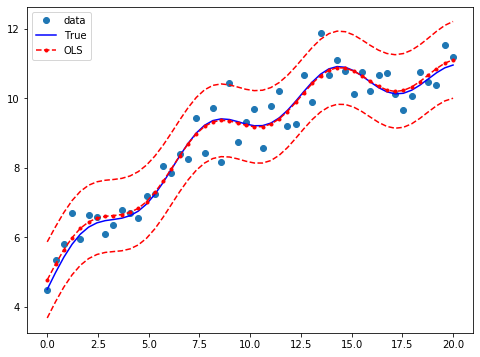3.2 广义线性模型——GLM
参考：https://www.statsmodels.org/stable/examples/notebooks/generated/glm.html
import statsmodels.formula.api as smf
star98 = sm.datasets.star98.load_pandas().data
formula = 'SUCCESS ~ LOWINC + PERASIAN + PERBLACK + PERHISP + PCTCHRT + \
PCTYRRND + PERMINTE*AVYRSEXP*AVSALK + PERSPENK*PTRATIO*PCTAF'
dta = star98[['NABOVE', 'NBELOW', 'LOWINC', 'PERASIAN', 'PERBLACK', 'PERHISP',
'PCTCHRT', 'PCTYRRND', 'PERMINTE', 'AVYRSEXP', 'AVSALK',
'PERSPENK', 'PTRATIO', 'PCTAF']].copy()
endog = dta['NABOVE'] / (dta['NABOVE'] + dta.pop('NBELOW'))
del dta['NABOVE']
dta['SUCCESS'] = endog

mod1 = smf.glm(formula=formula, data=dta, family=sm.families.Binomial()).fit()
mod1.summary()
mod1.predict(dta)


formula是常规的公式，其中所有X/Y数据都放在一个dataframe之中。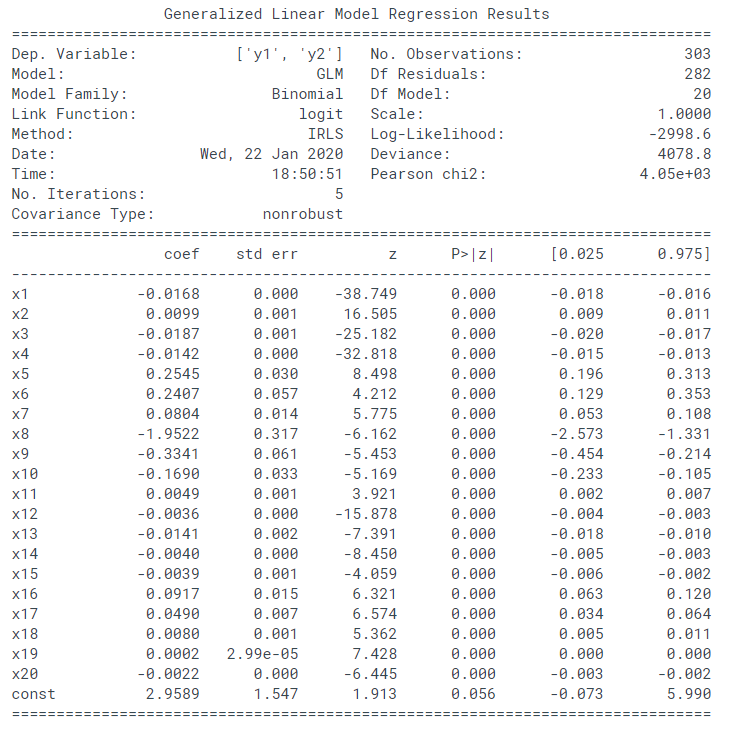print('Total number of trials:',  data.endog.sum())
print('Parameters: ', res.params)
print('T-values: ', res.tvalues)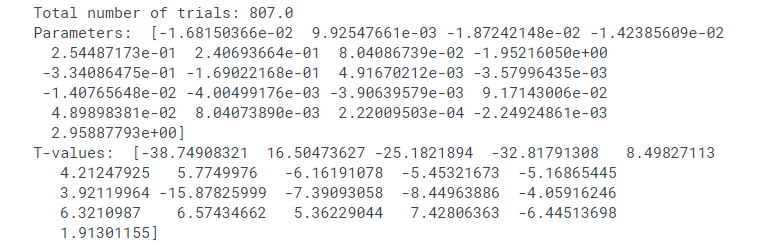包括了回归系数，T检验值
3.3 稳健回归
参考：https://www.statsmodels.org/stable/examples/notebooks/generated/robust_models_0.html
nsample = 50
x1 = np.linspace(0, 20, nsample)
X = np.column_stack((x1, (x1-5)**2))
X = sm.add_constant(X)
sig = 0.3   # smaller error variance makes OLS<->RLM contrast bigger
beta = [5, 0.5, -0.0]
y_true2 = np.dot(X, beta)
y2 = y_true2 + sig*1. * np.random.normal(size=nsample)
y2[[39,41,43,45,48]] -= 5   # add some outliers (10% of nsample)

X2 = X[:,[0,1]]
res2 = sm.OLS(y2, X2).fit()
print(res2.params)
print(res2.bse)

resrlm2 = sm.RLM(y2, X2).fit()
print(resrlm2.params)
print(resrlm2.bse)
print(resrlm2.summary())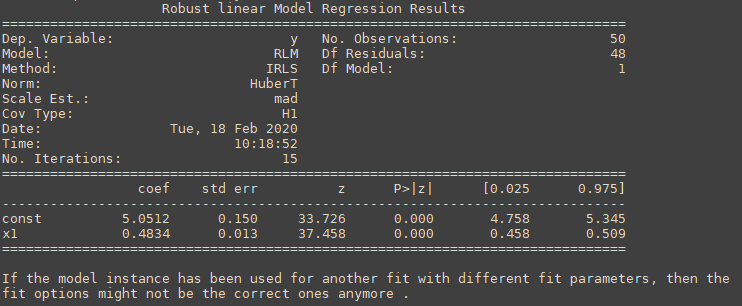4 其他
4.1 模型结果如何CSV导出？
可以通过as_csv()将模型导出
resrlm2 = sm.RLM(y, x).fit()
resrlm2.summary()
with open( 'model_rlm.csv', 'w') as fh:
fh.write(resrlm2.summary().as_csv())

不过导出的格式比较奇怪：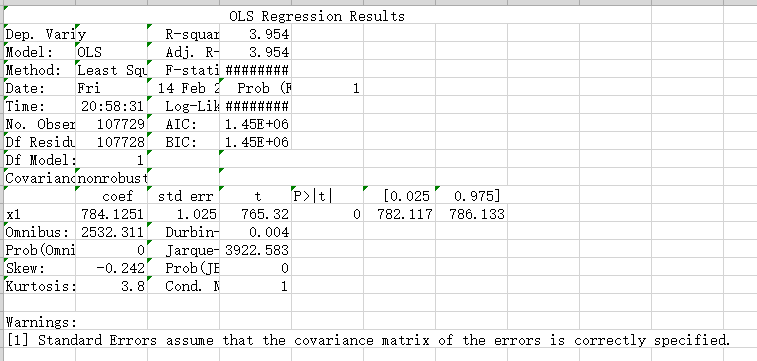4.2 画模型图以及保存
import statsmodels.api as sm
import numpy as np
import matplotlib.pyplot as plt

# 准备数据
x = np.linspace(0,10,100)
y = 3*x + np.random.randn()+ 10

# Fit and summarize OLS model
res = sm.OLS(y,x).fit()
print(res.params)
print(res.summary())
# 稳健回归
resrlm = sm.RLM(y, x).fit()

# 画图
fig, ax = plt.subplots(figsize=(8,6))
ax.plot(x, y, 'o', label="truey ")
ax.plot(x, res.predict(), 'o', label="ols")  # res2.predict(X2) == res2.predict()
ax.plot(x, resrlm.predict(), 'b-', label="rlm")# resrlm2.predict(X2) == resrlm2.predict()
legend = ax.legend(loc="best")

# 图保存
plt.savefig( 'image.jpg')


4.3 快速获取模型输出参数：P检验、F检验、P统计量
def get_model_param(res2,name = 'all'):
model_param_dict = {'name':name,  # 模型的名字
'rsquared':res2.rsquared, # R方
'fvalue':res2.fvalue, # F值，整个模型
'f_pvalue':res2.f_pvalue, # P值，整个模型
'params':res2.params, # 回归系数
'pvalues':res2.pvalues, # 回归系数 P检验 0.000
'tvalues':res2.tvalues} # 回归系数 T检验 276.571
return model_param_dict




展开全文机器学习
• ## python统计相关库

千次阅读 2017-11-23 23:25:25
from scipy import statsp=stats.poisson(10):以10为参数泊松分布； y=p.rvs(size=1000):对泊松分布进行1000点采样； plt.hist(y,bins=30,range=(0,30),color=’g’,normed=True):画图,bin表示是多少个直方，...
引入库：
from scipy import stats

p=stats.poisson(10):以10为参数的泊松分布；
y=p.rvs(size=1000):对泊松分布进行1000点采样；
plt.hist(y,bins=30,range=(0,30),color=’g’,normed=True):画图,bin表示是多少个直方，range指的是横坐标范围；
p.pmf(range(0,31))，得到possion的概率密度；
np.zeros(10)，生成10个全为0的数组；

待续。。。。就这些啦
展开全文• ## python统计函数库scipy.stats的用法1/3

万次阅读 多人点赞 2017-10-16 03:37:09
背景总结统计工作中几个常用用法在python统计函数scipy.stats使用范例。正态分布几个范例 生成服从指定分布随机数 norm.rvs通过loc和scale参数可以指定随机变量偏移和缩放参数，这里对应是正态分布...
背景

总结统计工作中几个常用用法在python统计函数库scipy.stats的使用范例。

正态分布

以正态分布的常见需求为例了解scipy.stats的基本使用方法。

生成服从指定分布的随机数
norm.rvs通过loc和scale参数可以指定随机变量的偏移和缩放参数，这里对应的是正态分布的期望和标准差。size得到随机数数组的形状参数。(也可以使用np.random.normal(loc=0.0, scale=1.0, size=None))

In : import numpy as np
In : import scipy.stats as st
In : st.norm.rvs(loc = 0,scale = 0.1,size =10)
Out:
array([ 0.12259875,  0.07001414,  0.11296181, -0.00630321, -0.04377487,
0.00474487, -0.00728678,  0.03860256,  0.06701367,  0.03797084])
In :
In : st.norm.rvs(loc = 3,scale = 10,size=(2,2))
Out:
array([[-13.26078265,   0.88411923],
[  5.14734849,  17.94093177]])
In :

求概率密度函数指定点的函数值
stats.norm.pdf正态分布概率密度函数。

In : st.norm.pdf(0,loc = 0,scale = 1)
Out: 0.3989422804014327
In : st.norm.pdf(np.arange(3),loc = 0,scale = 1)
Out: array([ 0.39894228,  0.24197072,  0.05399097])
In :

求累计分布函数指定点的函数值
stats.norm.cdf正态分布累计概率密度函数。

In : st.norm.cdf(0,loc=3,scale=1)
Out: 0.0013498980316300933
In : st.norm.cdf(0,0,1)
Out: 0.5
In :

累计分布函数的逆函数

stats.norm.ppf正态分布的累计分布函数的逆函数，即下分位点。

In : z05 = st.norm.ppf(0.05)
In :
In : z05
Out: -1.6448536269514729
In : st.norm.cdf(z05)
Out: 0.049999999999999975
In :

通用函数

stats连续型随机变量的公共方法：

名称
备注
rvs
产生服从指定分布的随机数
pdf
概率密度函数
cdf
累计分布函数
sf
残存函数（1-CDF）
ppf
分位点函数（CDF的逆）
isf
逆残存函数（sf的逆）
fit
对一组随机取样进行拟合，最大似然估计方法找出最适合取样数据的概率密度函数系数。
*离散分布的简单方法大多数与连续分布很类似，但是pdf被更换为密度函数pmf。

常见分布

可能用到的分布对照表

名称
含义
beta
beta分布
f
F分布
gamma
gam分布
poisson
泊松分布
hypergeom
超几何分布
lognorm
对数正态分布
binom
二项分布
uniform
均匀分布
chi2
卡方分布
cauchy
柯西分布
laplace
拉普拉斯分布
rayleigh
瑞利分布
t
学生T分布
norm
正态分布
expon
指数分布

参考文档

用Python做统计分析 （Scipy.stats的文档） - python论坛 - 经管之家(原人大经济论坛)
Scipy教程 - 统计函数库scipy.stats - 皮皮blog - CSDN博客
scipy.stats.weibull_max — SciPy v0.19.1 Reference Guide

展开全文numpy scipy stats
• 本次学习numpy库的统计相关操作知识，并对axis进行区分。numpy
• Python 中常用的统计工具有 Numpy, Pandas, PyMC, StatsModels 等。 Scipy 中的子 scipy.stats 中包含很多统计上的方法。 下面是scipy主要的模块，但用的最多的是stats cluster 聚类算法 constants 物理数学常数 ...数据分析
• /usr/bin/pythonimport os,sys,pymysqlmysqlbase = '/usr/local/mysql/bin/'if len(sys.argv) == 1: print('format: python + Script_name + user + password + ip')elif sys.argv == '-h': print('for......

# python的统计库python 订阅